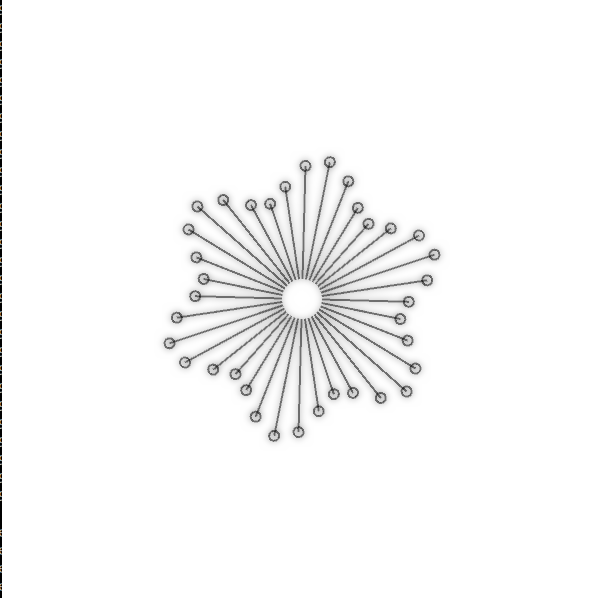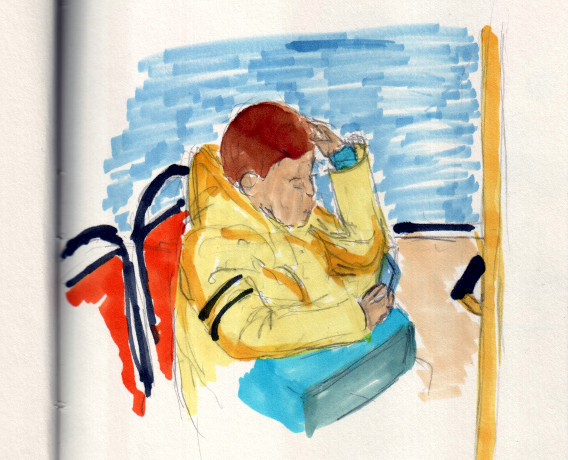## sketch experiment 8 - circles animated

for this sketch experiment I animated the circles i create in experiment 3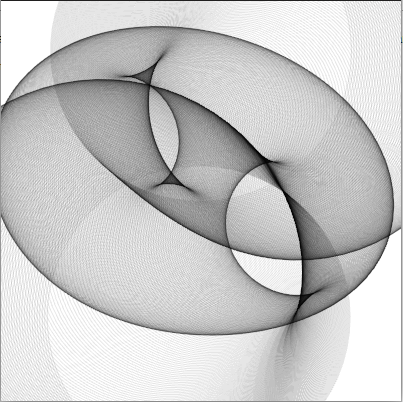``````(make-instance 'blub )
(defsketch blub ((title "circles anim") (p 0))
(background (rgb 1 1 1 ))
(incf p 0.1)
(translate 200 50)
(dotimes (i 720)
(with-pen (make-pen :stroke (rgb 0 0 0 0.1 ))
(push-matrix)

(setf f (+ p  (* (sin (* 6.28 (/ i 720)) ) (+ 0 (* 100 (cos (/ i 72.3)))))))
(circle (* 100 (sin (* 3.14 (/ i 180)))) (/ i 2.7) f)
(pop-matrix )
)
)
)
``````

## sketch experiment 7 - osc events

for my latest sketch experiment I implemented a osc event receiver that changes the size of a bouncing rectangle. The osc events are sent by a processing sketch.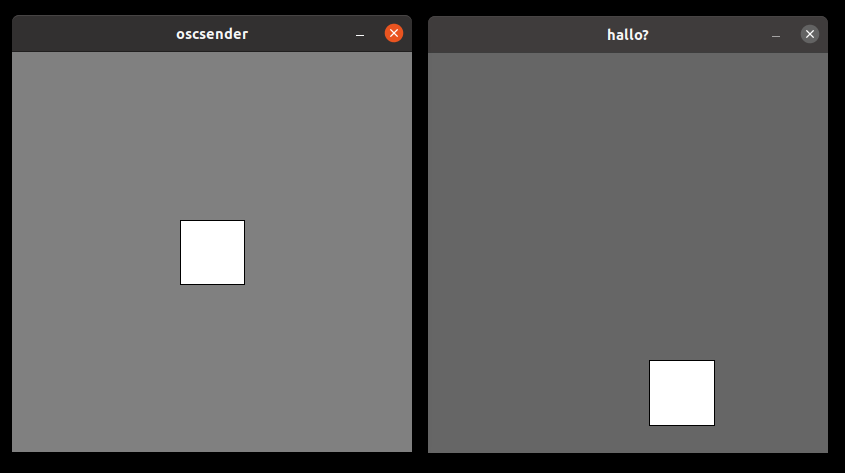## sketch experiment 6 - color

For my next sketch experiment I played with the hsb command to create animated colors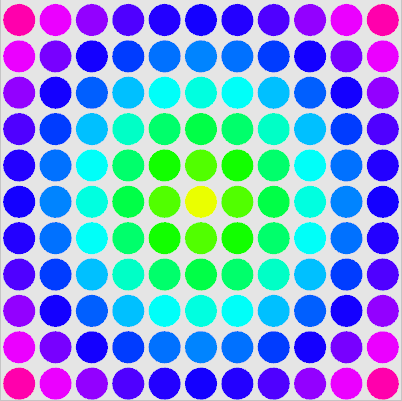What is a litte bit wired in sketch is how the sketch width and height affect the window size - i originally planned to resize the window to fit 11 circles each 40 pixel in diameter - but the only windowsize where the drawing area is centered seems to be 400 - not yet sure why - i will try to dig into the sourcecode of the sketch macro and figure out what happens here

here is the source code of my experiment

``````(defsketch blub (
(title "sketch ex6")
(width 400)
(height 400)

(f 0)
(p 0)
(w (/ 400 11))
)
(incf f 0.01)
(background (rgb .9 .9 .9))

(dotimes (i 11)
(dotimes (j 11 )
(setf dx (- 5 i))
(setf dy (- 5 j))
(if (> f 1) (setf f (- f 1)))

(setf p (+ f (* .1 (sqrt (+ (* dx dx) (* dy dy))))))
(if (> p 1) (setf p (ffloor p)))

(push-matrix)
(translate (+ (/ w 2) (* i w)) (+ 20 (* j w)))
(with-pen (make-pen :fill (hsb p 1 1))
(ellipse 0 0 (- (/ w 2) 2) (- (/ w 2) 2) ))
(pop-matrix)
)
)
)
``````

## sketch experiment 5 - grid

For my next sketch experiment I placed some rectangles on a grid and rotated them but delayed the rotation by the distance of the center.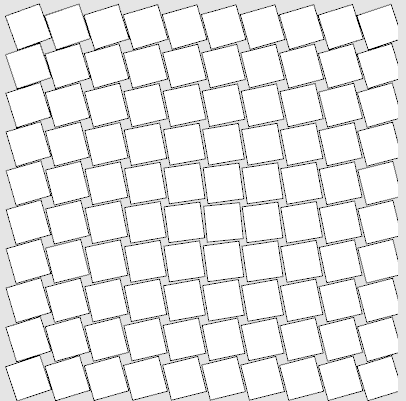this is the code I used to create the sketch - I probably should have transofrmed the distance calculation into a function now that I think of it. well - maybe next time :-)

``````(defsketch blub (
(title "sketch ex5")
(width 410)
(height 410)
(f 0)
(p 0)
)
(incf f 0.1)
(background (rgb .9 .9 .9))

(dotimes (i 10)
(dotimes (j 10 )
(setf dx (- 5 i))
(setf dy (- 5 j))
(setf p (- f (* 2 (sqrt (+ (* dx dx) (* dy dy))))))
(push-matrix)
(translate (+ 30 (* i 40)) (+ 25 (* j 40)))
(rotate p)
(rect -19 -19 36 36)
(pop-matrix)
)
)
)
``````

## sketch experiment 3 - animation

For experiment number 4 of my exploration of the commonlisp based sketch framewor, I started working with animations.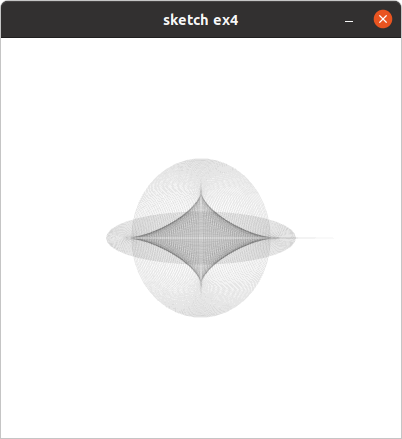obviously my previous sketches have been animations as well - they just drew the same picture at every frame.

``````(defsketch blub (
(title "sketch ex4")
(width 400)
(height 400)
(f 0)
(p 0)
)
(incf f 0.1)
(background (rgb 1 1 1))
(dotimes (i 100)
(setf p (- f (/ i 5)))
(with-pen (make-pen :stroke (rgb 0 0 0 0.1))
(ellipse (/ width 2) (/ height 2)
(* 100 (sin (/ p 10))) (* 100 (cos (/ p 17))))
)
)
)
``````

## sketch experiment 3 - circles

my third sketch experiment is using a similar technique I used in a previous ronin example - drawing a lot of circles along a curved path with a very transparent pen.

Its still a static image that gets rendered - I din't experiment with animations yet, but its very high on my todolist.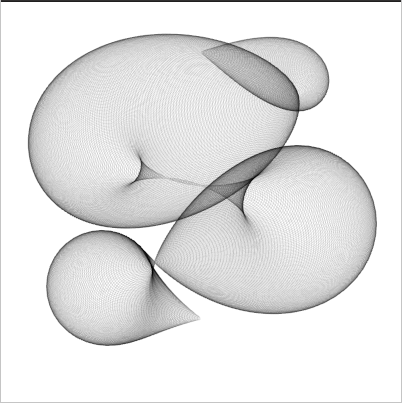``````(make-instance 'blub )
(defsketch blub ()
(background (rgb 1 1 1))
(translate 200 50)
(dotimes (i 720)
(with-pen (make-pen :stroke (rgb 0 0 0 0.1 ))
(push-matrix)

(setf f (* (sin (* 6.28 (/ i 720)) ) (+ 0 (* 100 (cos (/ i 72.3))))))
(circle (* 100 (sin (* 3.14 (/ i 180)))) (/ i 2.7) f)
(pop-matrix )
)
)
)
``````

## sketch experiment 2 - affine transformation

I continued my exploration of lisp-country with sketch and made a sketch experimenting with loops and affine transformations.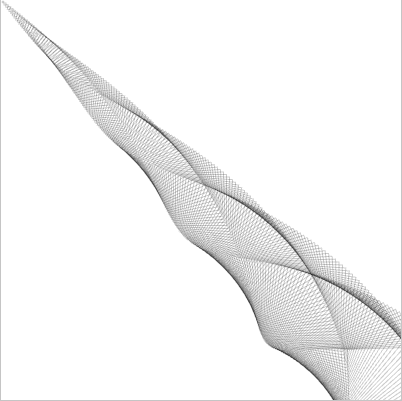that's the sketch that generates the image.

(make-instance 'blub ) (defsketch blub () (background (rgb 1 1 1)) (dotimes (i 200) (with-pen (make-pen :stroke (rgb 0 0 0 0.2 )) (push-matrix) (translate ( i 2) ( i 2)) (rotate (/ (* i 360) 200)) (setf f (/ i 2)) (rect (- (/ f 2)) (- (/ f 2)) f f ) (pop-matrix ) ) ) )

The documentation is a bit thin at the moment - but the sourcecode is quite readable, and there are some example programs I used as a starting point to figure out how to use it.

## sketch experiment 1 - openlisp based media art framework

Today I used sketch for the first time. It's a commonlisp based media art framework similar to processing. I haven't made something fancy yet, but so far I like it.

I also installed the vim plugin vlime for the first time - but I'm not yet sure if I really like it - maybe I need to use it for a little while to get used to it.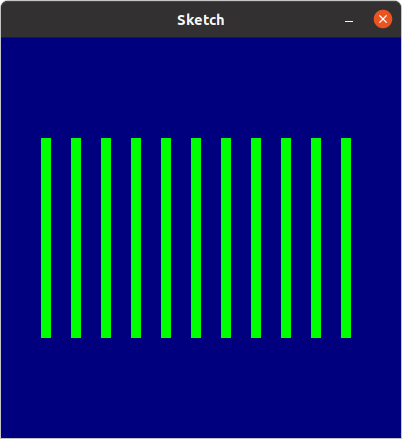the graphic was created using this lisp code

(defsketch boxes () (background (rgb 0 0 0.5)) (dotimes (i 11)
(with-pen (make-pen :fill (rgb 0 1 0)) (rect (+ (* i 30) 40) 100 10 200) ) ) )

## processing phaseflower

Since I haven't used Processing in a while, I dusted of my processing installation (and upgraded it to the latest version) and started to make some animations. I wanted to create an animation that morphes between random states of a parameterset over a random time periode, then stay at that state for some time and repeat ad infinitum. I implemented a similar state morphing system for a fractal in ronin a while ago and wanted to port it to processing. what could go wrong - right?

The base sketch I wanted to morph was a flower like shape that uses polar coordinates to modulate the radius of a bunch of small circles that are placed in a circle. Each target state that is held for some time should be a whole number multiple of the radius so that the modulations fit on a circle wihout jumps.

I implemented the drawing function and a first very simple state morphing system that is a direct port from my ronin sketch. There I simply interpolate between two states of a parameter over a given time

p = p1 + currenttime(p2 - p1)/periode

well that worked ok for the fractal curves i used id for in my last sketch, but since I used it here to do some modulations on a circular shape using polar coordinates the shape started to look really ugly when my parameter had some inbetween states that results in a fractual number. Hrmpf.

so back to the drawing board. Directly morphing the parameters and then calculating the position of the circles didn't work as expected.

After some experimenting I ended up at linear interpolation of the resulting circle positions that are calculated from the parametersets instead of morphing the paramters themslef. This way i can always use whole numbers as start and end values for a transition and no inbetween states that result in hard jumps are generated

lessions learned: simply morphing between states by blending parameters is fine in cartesian space - but results in wired transitions when polar coordinates or rotations are involved.

If you want to experiment with my state changing phase flower you can run it here.